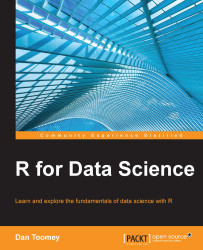•#### R for Data Science#### Overview of this book

R for Data ScienceCreditswww.PacktPub.comPrefaceFree Chapter
Data Mining PatternsData Mining SequencesText MiningData Analysis – Regression AnalysisData Analysis – CorrelationData Analysis – ClusteringData Visualization – R GraphicsData Visualization – PlottingData Visualization – 3DMachine Learning in ActionPredicting Events with Machine LearningSupervised and Unsupervised LearningIndex## Packages

In R, there are several packages available that provide the programmer with the regression functionality. We will be using the following packages in the examples:

• `chemometrics`: This package has tools to analyze chemometric data (multivariate)

• `MASS`: This package offers modern applied statistics with S

### Simple regression

In simple regression, we try to determine whether there is a relationship between two variables. It is assumed that there is a high degree of correlation between the two variables chosen for use in regression.

For this section, we will be using the `iris` dataset. The `iris` dataset has observations of the different characteristics of iris plants. For regression, we are seeing if there is a relationship between one characteristic of iris plants and others. As mentioned, the characteristics tested will have a high degree of correlation. The `iris` dataset is as follows:

`> data <- read.csv("http://archive.ics.uci.edu/ml/machine-learning-databases/iris/iris.data")`

Let's also...